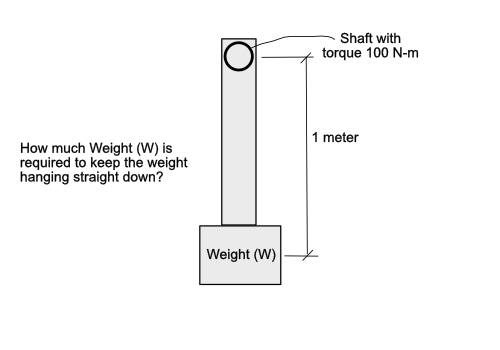# Weight required to hang straight down with known torque

• I
• MoMan

#### MoMan

How much Weight (W) is required to keep a weight hanging straight down when the torque is known on a rotating shaft? Please see attached image. Assume a lever weight of zero to keep it simple.Last edited:
•Delta2

it simple

Yeah, ##\infty##

••russ_watters, nasu, Lnewqban and 2 others
How much Weight (W) is required to keep a weight hanging straight down when the torque is known on a rotating shaft? Please see attached image. Assume a shaft weight of zero to keep it simple.View attachment 303451
Suppose that the shaft is deflected so that it does not hang straight down. Suppose that it is deflected by ##x## meters rightward, for instance. Do you know how to calculate how much restoring torque results from gravity pulling on the deflected weight?

There is a simple answer to the question you ask. But the Physics Forums way is to guide you into discovering that answer for yourself.

•Delta2
What's the application ?

Last edited:
•russ_watters
The way to answer this question with infinities: The torque of weight around the central point of the shaft is ##T_W=W\cdot 1m\cdot \sin 0##. For any finite W this equals to zero and hence the total torque will be equal to the torque from shaft and it will rotate the weight.
However if ##W=\infty## then $$T_W=\infty\cdot 0=\text {maybe something finite and equal to -}T_{shaft}$$ and hence for infinite weight the system might not rotate.

•Lnewqban
What's the application ?
It's either a puzzle (and not a very good one, IMO), or he's just trolling.

•Delta2 and jbriggs444
At what point do macro scale accelerations become imperceptible? Can we not effectively consider the rotation of ##0.1 ^{\circ}## over 10 years as effectively no acceleration. We do this kind of thing all the time in our modeling.

At what point do macro scale accelerations become imperceptible? Can we not effectively consider the rotation of ##0.1 ^{\circ}## over 10 years as effectively no acceleration. We do this kind of thing all the time in our modeling.
Well after the point at which suspended weights break the mechanism.

••Delta2 and erobz
$$m=\frac\tau{\mu_s{rg}}$$

Last edited: